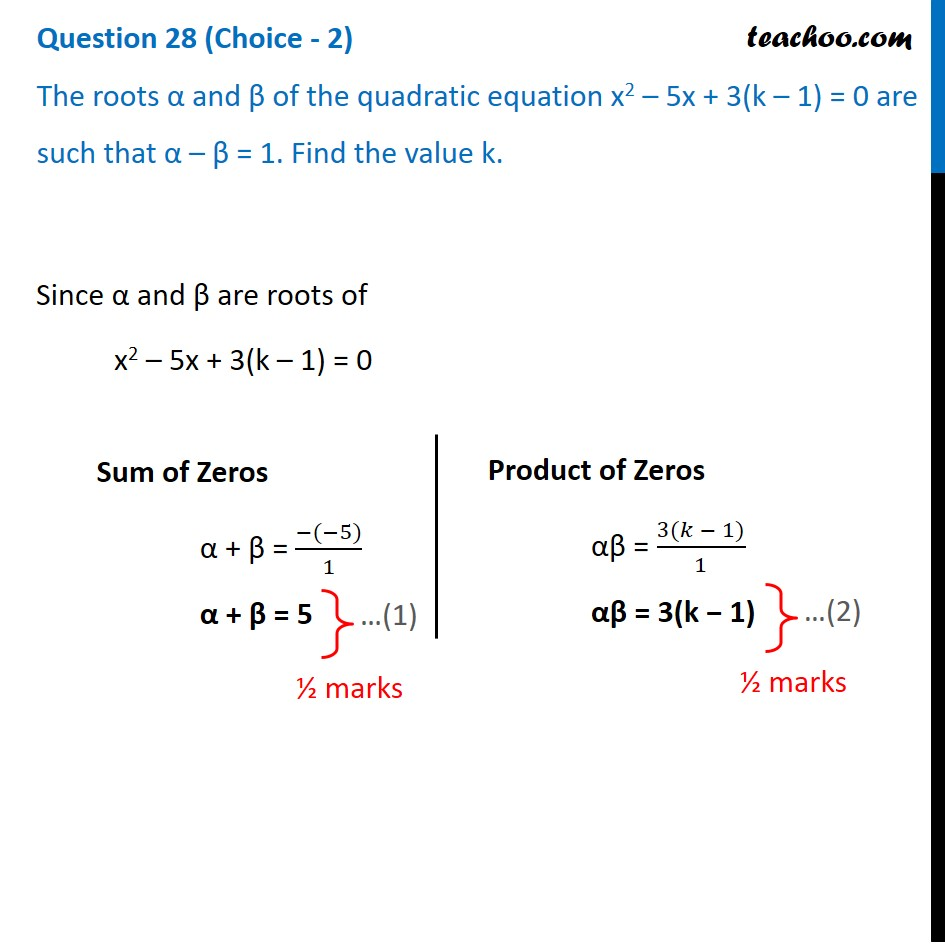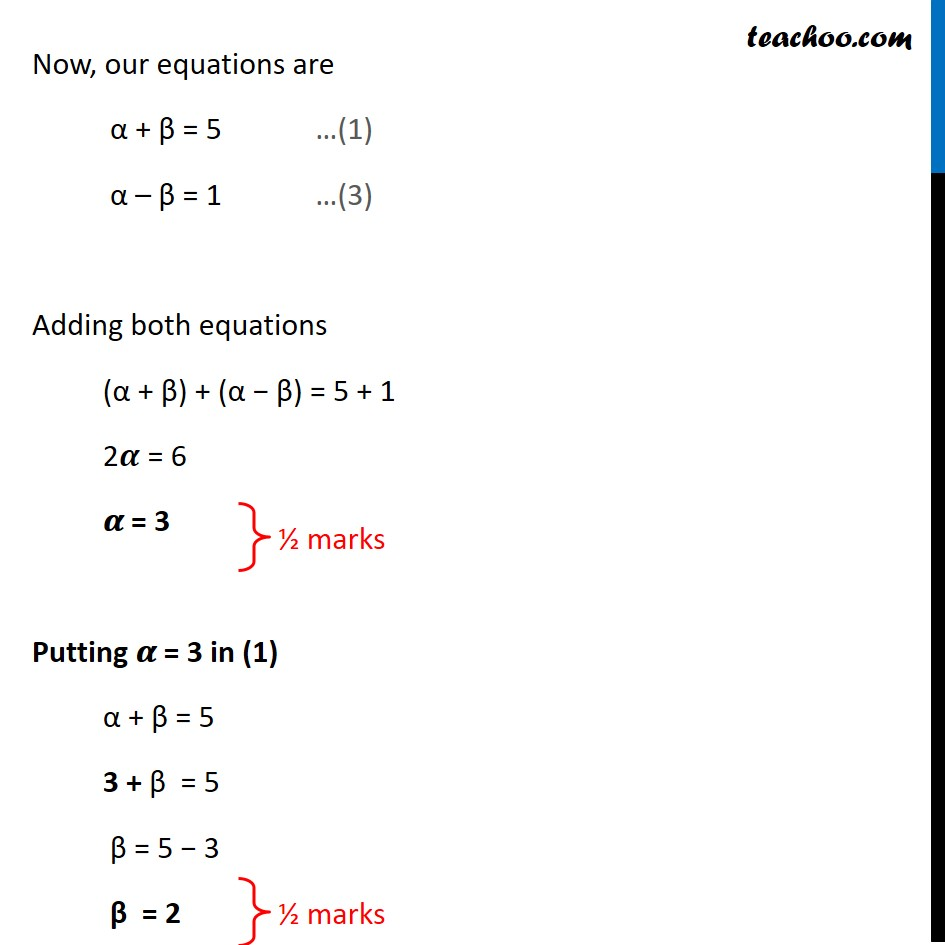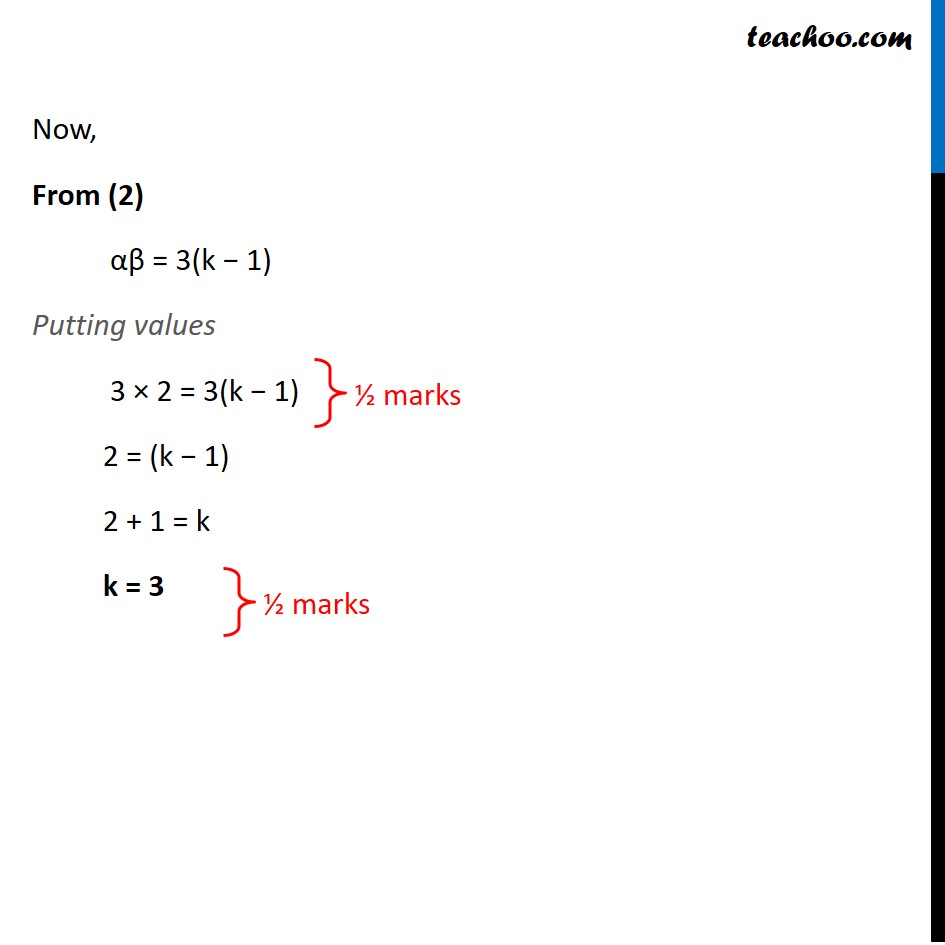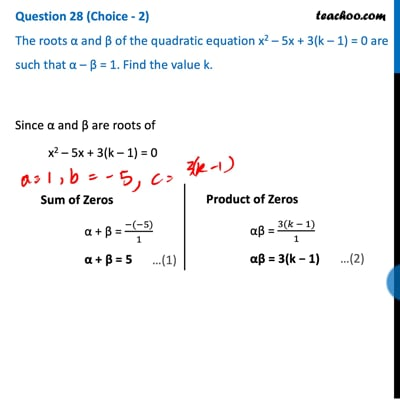CBSE Class 10 Sample Paper for 2021 Boards - Maths Standard

Class 10
Solutions of Sample Papers for Class 10 Boards

## The roots α and β of the quadratic equation x 2 – 5x + 3(k – 1) = 0 are such that α – β = 1. Find the value k.This video is only available for Teachoo black users

Introducing your new favourite teacher - Teachoo Black, at only ₹83 per month

### Transcript

Question 28 (Choice - 2) The roots α and β of the quadratic equation x2 – 5x + 3(k – 1) = 0 are such that α – β = 1. Find the value k.Since α and β are roots of x2 – 5x + 3(k – 1) = 0 Sum of Zeros α + β = (−(−5))/1 α + β = 5 Product of Zeros αβ = (3(𝑘 − 1))/1 αβ = 3(k − 1) Now, our equations are α + β = 5 …(1) α – β = 1 …(3) Adding both equations (α + β) + (α − β) = 5 + 1 2𝜶 = 6 𝜶 = 3 Putting 𝜶 = 3 in (1) α + β = 5 3 + β = 5 β = 5 − 3 β = 2 Now, From (2) αβ = 3(k − 1) Putting values 3 × 2 = 3(k − 1) 2 = (k − 1) 2 + 1 = k k = 3